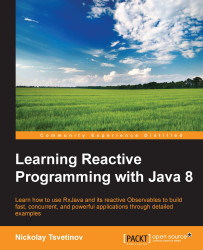•#### Learning Reactive Programming with Java 8#### Overview of this book

Learning Reactive Programming with Java 8Creditswww.PacktPub.comPrefaceFree Chapter
An Introduction to Reactive ProgrammingUsing the Functional Constructions of Java 8Creating and Connecting Observables, Observers, and SubjectsTransforming, Filtering, and Accumulating Your DataCombinators, Conditionals, and Error HandlingUsing Concurrency and Parallelism with SchedulersResource Management and Extending RxJavaIndex## What is reactive programming?

Reactive programming is a paradigm that revolves around the propagation of change. In other words, if a program propagates all the changes that modify its data to all the interested parties (users, other programs, components, and subparts), then this program can be called reactive.

A simple example of this is Microsoft Excel. If you set a number in cell A1 and another number in cell 'B1', and set cell 'C1' to `SUM(A1, B1)`; whenever 'A1' or 'B1' changes, 'C1' will be updated to be their sum.

Let's call this the reactive sum.

What is the difference between assigning a simple variable c to be equal to the sum of the a and b variables and the reactive sum approach?

In a normal Java program, when we change 'a' or 'b', we will have to update 'c' ourselves. In other words, the change in the flow of the data represented by 'a' and 'b', is not propagated to 'c'. Here is this illustrated through source code:

```int a = 4;
int b = 5;
int c = a + b;
System.out.println(c); // 9

a = 6;
System.out.println(c);
// 9 again, but if 'c' was tracking the changes of 'a' and 'b',
// it would've been 6 + 5 = 11```